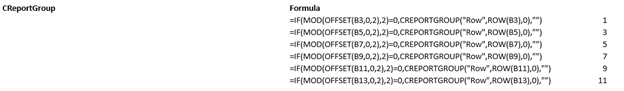# CREPORTGROUP¶

CREPORTGROUP only functions in published reports - so while the formula is entered in Excel, the behaviour only becomes evident after the report is published.

CREPORTGROUP is used to provide similar functionality as “grouping cells” in Excel but in a way that can be interpreted by a published report.

When combined with conditional Excel formulas (such as `IF`) this formula becomes dynamic.

Syntax

CREPORTGROUP (RowOrCol, Start, Offset)

### Arguments¶

#### RowOrCol : `string`(required)¶

“Row” or “Col” (case insensitive and flexible - ie all the following are valid: “column”, “col”, “COL”, “Col”)

#### Start : `string`¶

The row or column to start the group from. A letter should be used for columns and numbers should be used for rows.

The default start is the row/col of the owning formula cell.

#### Offset : `number`¶

The number of rows or columns to include in the group from the starting cell defined in Start. This should be a number if using “Row”.

The default offset is 0.

## Usage Scenarios¶

Report groups are good for hiding rows and columns where the rows and columns to be hidden may change during a calculation. When combined with Excel IF formulas,  you can make this formula dynamic. Note if using any MDX based query to recall values, the conditional IF formula should also utilize the Excel OFFSET formula

## Examples¶

To hide rows 10 to 15, the following formula would be entered (it can be on any non data cell, in this instance it has been entered into cell A4):

`=CREPORTGROUP("ROW",10,5)`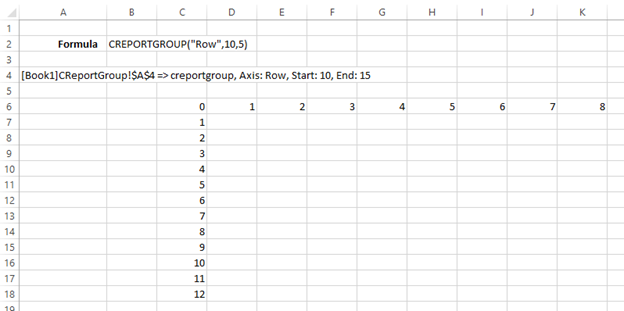When published, the following is displayed in the web (Rows 10 to 15 inclusive are hidden):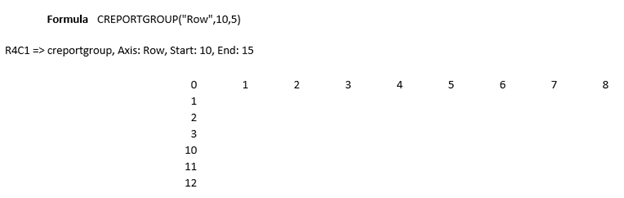Changing to columns, to hide columns E to J, the following would be entered:

`=CREPORTGROUP("COL","E",5) or =CREPORTGROUP("COLUMN","E",5)`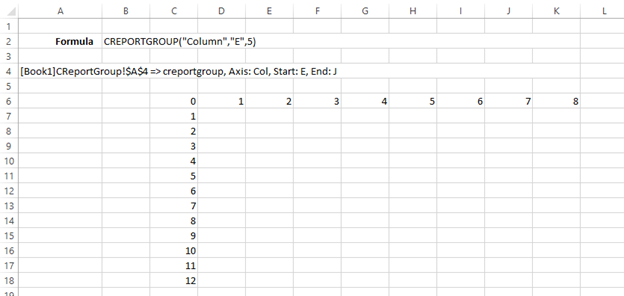And when published: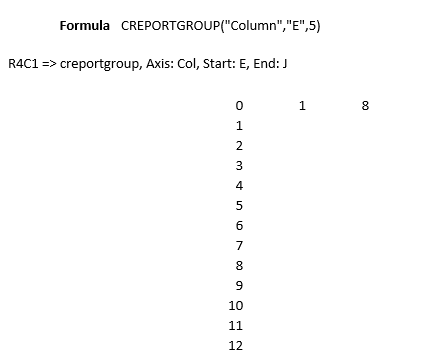Using the Excel IF function, we can make the formula dynamic. If we wanted to hide every 2nd row using the MOD and IF functions, we can only apply the CALUMO CREPORTGROUP function whenever Mod(Row,2) = 0. In Excel it appears as:On publishing, it appears as: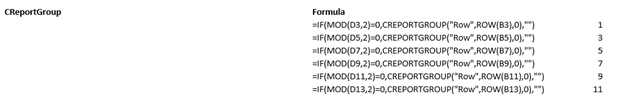With all even rows hidden. To modify the query to use the Excel OFFSET function (if data comparison was MDX based), the formula would be altered as illustrated: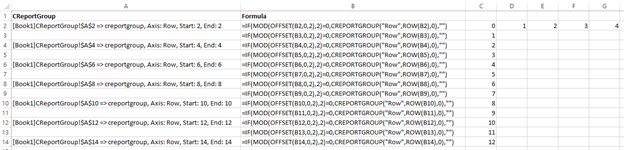Publishing still provides similar output. In a production environment formula rows and columns would be hidden.